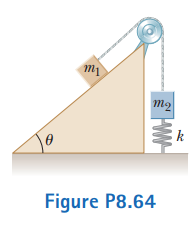# Problem: A block of mass m1 = 20.0 kg is connected to a block of mass m2 = 30.0 kg by a massless string that passes over a light, frictionless pulley. The 30.0-kg block is connected to a spring that has negligible mass and a force constant of k = 250 N/m as shown in the figure. The spring is unstretched when the system is as shown in the figure, and the incline is frictionless. The 20.0-kg block is pulled a distance h = 20.0 cm down the incline of angle θ = 40.0° and released from rest. Find the speed of each block when the spring is again unstretched.

###### FREE Expert Solution
94% (369 ratings)
###### Problem Details

A block of mass m1 = 20.0 kg is connected to a block of mass m2 = 30.0 kg by a massless string that passes over a light, frictionless pulley. The 30.0-kg block is connected to a spring that has negligible mass and a force constant of k = 250 N/m as shown in the figure. The spring is unstretched when the system is as shown in the figure, and the incline is frictionless. The 20.0-kg block is pulled a distance h = 20.0 cm down the incline of angle θ = 40.0° and released from rest. Find the speed of each block when the spring is again unstretched.Frequently Asked Questions

What scientific concept do you need to know in order to solve this problem?

Our tutors have indicated that to solve this problem you will need to apply the Energy in Connected Objects (Systems) concept. You can view video lessons to learn Energy in Connected Objects (Systems). Or if you need more Energy in Connected Objects (Systems) practice, you can also practice Energy in Connected Objects (Systems) practice problems.

How long does this problem take to solve?

Our expert Physics tutor, Julia took 6 minutes and 28 seconds to solve this problem. You can follow their steps in the video explanation above.

What professor is this problem relevant for?

Based on our data, we think this problem is relevant for Professor Jerousek's class at UCF.

What textbook is this problem found in?

Our data indicates that this problem or a close variation was asked in Physics for Scientists and Engineers - Serway Calc 9th Edition. You can also practice Physics for Scientists and Engineers - Serway Calc 9th Edition practice problems.